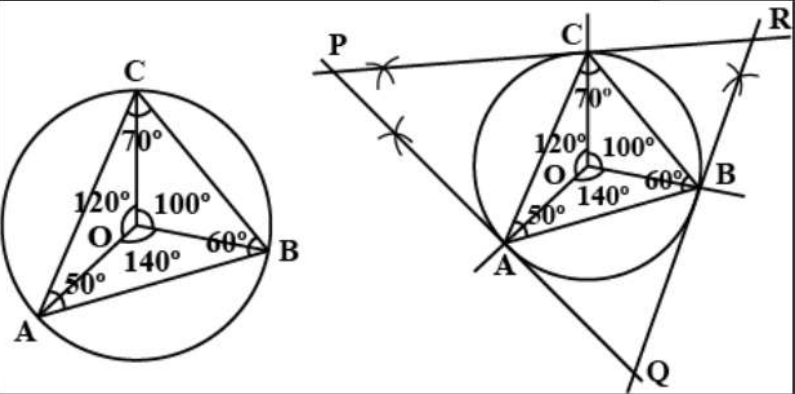Courses
Courses for Kids
Free study material
Free LIVE classes
MoreLIVE
Join Vedantu’s FREE Mastercalss

# Draw a circle of radius $3cm$Draw triangle $ABC$ with this circle as circumcircle and angle ${50^ \circ },{60^ \circ }$ and ${70^ \circ }$.Construct triangle$PQR$, outside the circle, by drawing tangents to the circle at the points $A,B$and$C$.Find all angles of triangle$PQR$Verified
361.5k+ views
Hint: Use the necessary tools to attempt construction problems. Follow the construction steps.

Steps of Construction:

Step #1: Draw a circle with centre $O$ having radius $3cm$.
Step #2: Take three points $A,B$and $C$ in the circle and join $A$ to the centre of the circle $O$.
Step #3: If $\angle BAC$ is to be${50^ \circ }$, should be${100^ \circ }$.
Step #4: If $\angle ABC$ is to be${60^ \circ }$, should be${120^ \circ }$.
Step #5: If $\angle ACB$ is to be${70^ \circ }$, should be${140^ \circ }$.
Step #6: Join the points B and C such that $m\angle AOC = {100^ \circ }$ and$m\angle BOC = {120^ \circ }$.
Thus, $\Delta ABC$is the required triangle.
Steps of Construction:

Step #1: Extend $OA$ and draw perpendicular to it through$A$.
Step #2: Extend $OB$ and draw perpendicular to it through$B$.
Step #3: In the same way draw a perpendicular from point $C$ through$OC$.
Let the points of intersection of these perpendicular be $P,Q$ and$R$, so we get the required $\Delta PQR$.
In the quadrilateral $PAOC$,

$m\angle AOC = {120^ \circ }$
$\Rightarrow m\angle P = {180^ \circ } - {120^ \circ } = {60^ \circ }$ ……(opposite angles of a quadrilateral are supplementary)
In the same way,
$\Rightarrow m\angle Q = {180^ \circ } - {140^ \circ } = {40^ \circ }$
And, $\Rightarrow m\angle R = {180^ \circ } - {100^ \circ } = {80^ \circ }$Note: Use the necessary tools to attempt construction problems. Mistakes can be made in reading the angles wrongly on the instruments or incorrect dimensioning in the diagram.
Last updated date: 23rd Sep 2023
Total views: 361.5k
Views today: 10.61k